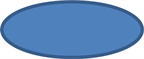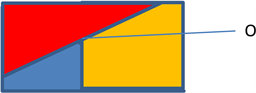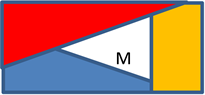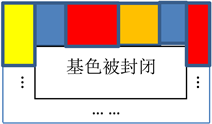﻿ 四色定理的数学证明

# 四色定理的数学证明Mathematical Proof of Four-Color Theorem

Abstract: The closed space is defined as an area, and then the background color of the map is defined. The section connecting more than three boundaries is regarded as the intersection point, and the line segment connecting two regions is defined as the boundary. After reducing or expanding the in-tersection points of the map, the complex area map with boundary and intersection points is sim-plified to a map with only boundary. The results show that four kinds of color can be used to dis-tinguish different areas in the map.

1. 预备工作Figure 1. A space enclosed by a single lineFigure 2. Odd line segmentFigure 3. Even line segmentFigure 4. Intersection of three regionsFigure 5. Base color is closed

2. 命题证明

1) 区域，2) 被封闭的基色区，3) 交点，4) 边界。Figure 6. After the intersection of n regions expands

NOTES

 Zhou, P.S. (2010) Computer Science. China Science and Technology Press, Beijing.

 Gu, C.H. (1992) Mathematics Dictionary. Shanghai Dictionary Press, Shanghai.

 Min, S.H. (1981) Method of Number Theory. Science Press, Beijing.

Top